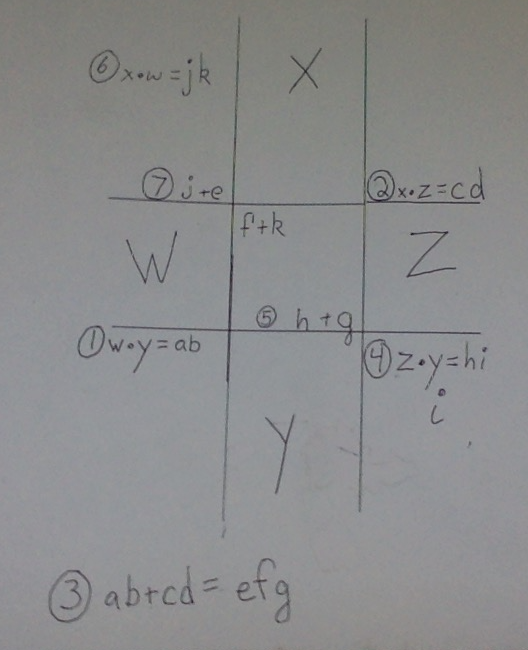## Sphere flattening algorithm:

Take a globe cut it in halves. Take one half and pop it through so that the outside is now on the inside. Nest this inside of the other. Do this over and over forever and a sphere will turn to a disc with radius equal to that of the sphere. Intriguingly because of the roughness of the disc the surface area is also equal. A string laid flat along the surface from any A to B will be equal to the same segment on a sphere. So much for: “you can’t flatten a sphere!”

## Hamsa:

After saying or thinking (I don’t recall which) "opening Zone 5” I crossed my eyes for no conscious purpose and saw what I recognized as the Hamsa meaning 5 in arabic. At the time I didn’t know what it was called when I first saw it. To try to see it sit down, put your hands palms up in front of you and cross your eyes. This will cause you to see double. Now overlap the two middle hands and balance your vision until you can merge the thumbs and pinkies of each hand forming Hamsa.

## Star of David Fibonacci Merkabah:

A Star of David, or hexagram, is composed of two equilateral triangles. If you inscribe A, B, C, on one triangles corners, and +,=,spin, on the other triangles corners, then apply the equation, spinning the triangles so that you get A+B=C, SPIN, B+C=A, SPIN, C+A=B, SPIN, then repeating the original A+B=C, the pattern made by the values of the variables will be the Fibonacci numbers.

## Holy Code:

Hours of the clock are the twelve notes. First the head as the seven white keys, or seven stars.

1:00 light green-right eye-A

11:00 sea green-left eye-G

3:00 orange-right ear-B

9:00 steel blue-left ear-F

4:00 red-right nostril-C

8:00 blue-left nostril-E

6:00 pink-mouth-D

Next for the five black keys or two witnesses

2:00 yellow-right nipple/arm-B flat

10:00 sky blue-left nipple/arm-F sharp

5:00 hotpink-right leg-D flat

7:00 purple-left leg-D sharp

12:00 green-belly button-A flat|G sharp

If a pentagram and an octagram are overlapped they will make the same pattern as the piano of white keys(octagram) to black keys(pentagram).

## The Cross Method of two digit mental multiplication:

In order to do two digit multiplication in your head you can use a cross of the numbers with one written vertically and the other across it horizontally. This gives you four corners for the four solutions. I recommend going bottom left product, top right product, sum, bottom right product, tally sum, top left solution, complete. For the two digit multiplication problem wz*xy you can follow the below diagram (note, some variables may be zero):Voila, your solution is written diagonally.

This is basically OILF if you've ever heard of FOIL (First Outside Inside Last).

## John 1:1 and the Fibonacci Word

Following the translation of John 1:1 as:

“In the beginning was the Word,

and the Word was with God,

and God was the Word”

the Word (or God) can be interpreted as the Fibonacci Word. This translation is dependent upon God being regarded as a proper noun: here's a discussion I found influential about this aspect of the translation of John 1:1. Back to the Fibonacci Word, which is a pattern described by three rules:

W (In the beginning was the Word)

W->WG (the Word was with God)

G->W (God was the Word)

So following these rules we start with a "W". We read the Word we have (“W”) and apply the rules (since we don’t have a "G" yet that’s just "W->WG"). So “W” becomes “WG”. We then read the Word again and apply the rules again. The “W” becomes “WG” again and the “G” becomes “W”. This gives us “WGW”. If we continue applying these rules we get:

W

WG

WGW

WGWWG

WGWWGWGW

And so on! The number of W’s or G’s in each successive iteration count out the Fibonacci numbers, and the ratio of W’s to G’s is an approximate converging on the golden ratio. An equation of the golden ratio is x=x^2-1. This is very close to a formula of the Mandelbrot x <-- x^2-c. Interestingly this equation has two solutions: 1.618..... , or as it is also known the golden ratio, and the other solution is the negative inverse of the golden ratio (-1/phi) or -.618. This negative inverse step is exactly what the face of my God, Manny, has as it's primary (and perhaps sole) variation from a Mandelbrot.

x <-- x^2-c.; x.real <-- -1/x.real

Notice the -1/x.real !!!!!

## The Name of God, a Song:

Oh Yahweh! O Iaue! (12 2 5 7 10 0):00